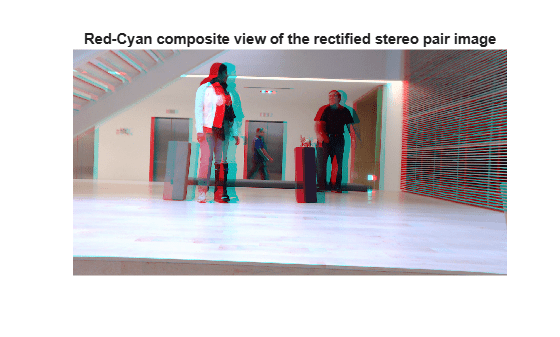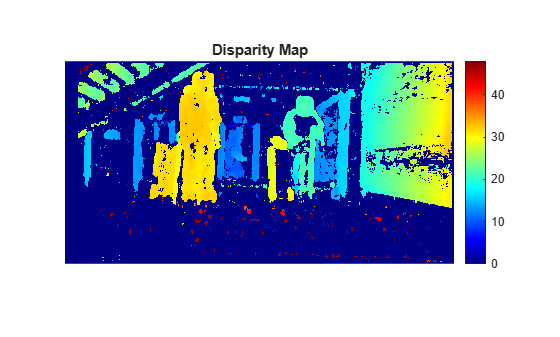# disparityBM

Compute disparity map using block matching

## Syntax

``disparityMap = disparityBM(I1,I2)``
``disparityMap = disparityBM(I1,I2,Name,Value)``

## Description

example

````disparityMap = disparityBM(I1,I2)` computes disparity map from a pair of rectified stereo images `I1` and `I2`, by using the block matching method. To know more about rectifying stereo images, see Image Rectification.```
````disparityMap = disparityBM(I1,I2,Name,Value)` specifies options using one or more name-value pair arguments.```

## Examples

collapse all

Load a rectified stereo pair image.

```I1 = imread('rectified_left.png'); I2 = imread('rectified_right.png');```

Create the stereo anaglyph of the rectified stereo pair image and display it. You can view the image in 3-D by using red-cyan stereo glasses.

```A = stereoAnaglyph(I1,I2); figure imshow(A) title('Red-Cyan composite view of the rectified stereo pair image')```Convert the rectified input color images to grayscale images.

```J1 = im2gray(I1); J2 = im2gray(I2);```

Compute the disparity map. Specify the range of disparity as [0, 48], and the minimum value of uniqueness as 20.

```disparityRange = [0 48]; disparityMap = disparityBM(J1,J2,'DisparityRange',disparityRange,'UniquenessThreshold',20);```

Display the disparity map. Set the display range to the same value as the disparity range.

```figure imshow(disparityMap,disparityRange) title('Disparity Map') colormap jet colorbar```## Input Arguments

collapse all

Input image referenced as `I1` corresponding to camera 1, specified as a 2-D grayscale image or a `gpuArray` (Parallel Computing Toolbox) object. The function uses this image as the reference image for computing the disparity map. The input images `I1` and `I2` must be real, finite, and nonsparse. Also, `I1` and `I2` must be of the same size and same data type.

Data Types: `single` | `double` | `int16` | `uint8` | `uint16`

Input image referenced as `I2` corresponding to camera 2, specified as a 2-D grayscale image or a `gpuArray` (Parallel Computing Toolbox) object. The input images `I1` and `I2` must be real, finite, and nonsparse. `I1` and `I2` must be of the same size and same data type.

Data Types: `single` | `double` | `int16` | `uint8` | `uint16`

### Name-Value Arguments

Specify optional pairs of arguments as `Name1=Value1,...,NameN=ValueN`, where `Name` is the argument name and `Value` is the corresponding value. Name-value arguments must appear after other arguments, but the order of the pairs does not matter.

Before R2021a, use commas to separate each name and value, and enclose `Name` in quotes.

Example: `disparityBM(I1,I2,'DisparityRange',[0 64])`

Range of disparity, specified as the comma-separated pair consisting of `'DisparityRange'` and a two-element vector of the form [MinDisparity MaxDisparity]. MinDisparity is the minimum disparity and MaxDisparity is the maximum disparity. The conditions this range must satisfy depend on the type of input images.

If the input images are grayscale images of width N, then:

• MinDisparity and MaxDisparity must be integers in the range (–N, N).

• The difference between the MaxDisparity and MinDisparity values must be divisible by 16 and less than the width of the input images.

If the input images are `gpuArray` objects of width N, then:

• The value of MinDisparity must be equal to zero.

The value of MaxDisparity must be in the range (16, N). If N is greater than 256, then the MaxDisparity must be chosen as less than or equal to 256.

The difference between the MaxDisparity and MinDisparity values must be divisible by 16.

The default value for the range of disparity is `[0 64]`. For more information on choosing the range of disparity, see Choosing Range of Disparity.

Size of the squared block, specified as the comma-separated pair consisting of `'BlockSize'` and an odd integer. This value specifies the width of the search window used for block matching pixels in the rectified stereo pair image. The range for the size squared block depend on the type of input images.

If the input images are grayscale images, the `'BlockSize'` value must be an odd integer in the range [5, 255].

If the input images are `gpuArray` objects, the `'BlockSize'` value must be an odd integer in the range [5, 51].

The default value is 15.

Range of contrast threshold, specified as the comma-separated pair consisting of `'ContrastThreshold'` and a scalar value in the range (0, 1]. The contrast threshold defines an acceptable range of contrast values. If the contrast value of a pixel in the reference image is below the contrast threshold, then the disparity computed for that pixel is considered unreliable. Increasing this parameter results in disparity for fewer pixels being marked as unreliable.

Minimum value of uniqueness, specified as the comma-separated pair consisting of `'UniquenessThreshold'` and a nonnegative integer.

The function marks the estimated disparity value K for a pixel as unreliable, if:

v < V×(1+0.01×`UniquenessThreshold`),

where V is the sum of absolute difference (SAD) corresponding to the disparity value K. v is the smallest SAD value over the whole disparity range, excluding K, K–1, and K+1.

Increasing the value of `UniquenessThreshold` results in disparity values for more pixels being marked as unreliable. To disable the use of uniqueness threshold, set this value to `[]`.

Note

If the input images are `gpuArray` objects, then the `'UniquenessThreshold'` name-value pair argument does not apply.

Maximum distance between conjugate pixels in stereo pair image, specified as the comma-separated pair consisting of `'DistanceThreshold'` and a nonnegative integer. The distance threshold specifies the maximum distance between a pixel in `I1` and the same pixel found in `I2`.

For pixel p1 in the reference image `I1`, the function performs a left-to-right check to find its best matching pixel p2 in image `I2`. For pixel p2, the function performs a right-to-left check to find its best matching pixel p3 in the reference image `I1`. If the distance between p1 and p3 is greater than the `DistanceThreshold`, the function marks the disparity for the pixel p1 in the reference image `I1` as unreliable.

Increasing the value of `DistanceThreshold` results in disparity values for fewer pixels being marked as unreliable. Conversely, decreasing the value of `DistanceThreshold`, increases the reliability of the disparity map. To disable the use of distance threshold, set this value to `[]`.

Note

If the input images are `gpuArray` objects, then the `'DistanceThreshold'` name-value pair argument does not apply.

Minimum texture threshold, specified as the comma-separated pair consisting of `'TextureThreshold'` and a scalar value in the range [0, 1).

The texture of a pixel is defined as the sum of the saturated contrast computed over the `BlockSize`-by-`BlockSize` window around the pixel. The texture threshold defines the minimum texture value for a pixel to have reliable disparity value. The function considers the disparity for a pixel as unreliable, if:

texture < intensitymax×`TextureThreshold`×`BlockSize`2,

where texture is the texture of a pixel. intensitymax is the maximum value supported by the data type of input images `I1` and `I2`.

Increasing the value of `'TextureThreshold'` results in disparity values for more pixels being marked as unreliable. To disable the use of texture threshold, set this value to `[]`.

## Output Arguments

collapse all

Disparity map for rectified stereo pair image, returned as a 2-D grayscale image or a `gpuArray` object. The function returns the disparity map with the same size as input images `I1` and `I2`. Each value in this output refers to the displacement between conjugate pixels in the stereo pair image. For details about computing the disparity map, see Computing Disparity Map Using Block Matching.

Data Types: `single`

collapse all

### Image Rectification

The input images `I1` and `I2` must be rectified before computing the disparity map. The rectification ensures that the corresponding points in the stereo pair image are on the same rows. You can rectify the input stereo pair image by using the `rectifyStereoImages` function. The reference image must be the same for rectification and disparity map computation.

## Algorithms

collapse all

### Choosing Range of Disparity

The range of disparity must be chosen to cover the minimum and the maximum amount of horizontal shift between the corresponding pixels in the rectified stereo pair image. You can determine the approximate horizontal shift values from the stereo anaglyph of the stereo pair image. Compute the stereo anaglyph of the rectified images by using the `stereoAnaglyph` function. Display the stereo anaglyph in the Image Viewer app. To measure the amount of horizontal shift between the corresponding points in the stereo pair image, select Measure Distance from the Tools menu in Image Viewer. Choose the minimum and maximum disparity values for the range of disparity based on this measurement.

For example, this figure displays the stereo anaglyph of a rectified stereo pair image and the horizontal shift values measured between the corresponding points in the stereo pair image. The minimum and maximum shift values are computed as 8 and 31 respectively. Based on these values, the range of disparity can be chosen as [0, 48].### Computing Disparity Map Using Block Matching

The function computes the disparity map by following these steps:

1. Compute the disparity for each pixel by using the block matching method given in .

• For CPU — The sum of absolute difference (SAD) is used as the cost function in block matching. The cost function estimates the displacement between pixels in the rectified stereo pair image.

2. Optionally, mark the pixels for unreliability based on the `ContrastThreshold`, `UniquenessThreshold`, `DistanceThreshold`, and `TextureThreshold` name-value pairs. The function sets the unreliable disparity values to `NaN`.

 Konolige, K. "Small Vision Systems: Hardware and Implementation." In Proceedings of the 8th International Symposium in Robotic Research, pp. 203–212. 1997.

 Bradski, G. and A. Kaehler. Learning OpenCV : Computer Vision with the OpenCV Library. Sebastopol, CA: O'Reilly Media, Inc. 2008.